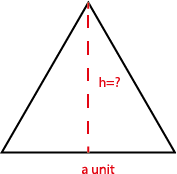Posts

Showing posts from November, 2018

The Percentage of A NumberTo find the percentage of any number;

Divide the number into 100 equal parts, (this way you can find out the value of its 1%)Multiply with the percentage you need (this way whatever % you need, you can calculate this)
What is 40% of 30?

The Area of An Equilateral TriangleThe way to find the area of an equilateral triangle is the same as finding the area of other triangles.

$$\text{The area of a triangle :}\frac{\text{Base x Base Height}}{2}$$

According to the above formula, we need to know the base and base height. However is it possible to calculate one or the other if we don’t know its value? For example, generally the base (of an equilateral triangle) is known but the base height is not known. The main problem is; can we know the height by using the base value?

First of all, let us look at the height of isosceles and equilateral triangles. Let us have a triangle where the base is equal but the arms can be flexible .

When both sides are equal then the height is exactly joint together in the middle point.

The yellow and blue lengths are equal, the green length, i.e. the height has split the base into two equals as shown.
Now our triangle is an isosceles triangle, if the black edge, i.e. the base has equal length as the other sides it becomes an equila…

Fraction of an amountTo find the fraction of a number / amount , or the exact opposite, to find the whole number of a fraction it is important to know unit fractions.

Fractions are made up of equal partsOne of the equal parts is called a unit fraction

Absolute ValueThe value that is created from the start point onwards is called absolute value.

To understand absolute value, let us start with a question.
The weather in Istanbul is twice as warm as it is in London. If it is 0° C. in London, what is the weather in Istanbul ?